# Maharashtra Board Class 12 Physics Solutions Chapter 4 Thermodynamics

Balbharti Maharashtra State Board 12th Physics Textbook Solutions Chapter 4 Thermodynamics Textbook Exercise Questions and Answers.

## Maharashtra State Board 12th Physics Solutions Chapter 4 Thermodynamics

1. Choose the correct option.

i) A gas in a closed container is heated with 10J of energy, causing the lid of the container to rise 2m with 3N of force. What is the total change in the internal energy of the system?
(A) 10J
(B) 4J
(C) -10J
(D) – 4J
(B) 4J

ii) Which of the following is an example of the first law of thermodynamics?
(A) The specific heat of an object explains how easily it changes temperatures.
(B) While melting, an ice cube remains at the same temperature.
(C) When a refrigerator is unplugged, everything inside of it returns to room temperature after some time.
(D) After falling down the hill, a ball’s kinetic energy plus heat energy equals the initial potential energy.
(B) While melting, an ice cube remains at the same temperature. [Here, ∆u = 0, W = Q]
(C) When a refrigerator is unplugged, everything inside of it returns to room temperature after some time.
(D) After falling down the hill, a ball’s kinetic energy plus heat energy equals the initial potential energy.iii) Efficiency of a Carnot engine is large when
(A) TH is large
(B) TC is low
(C) TH – TC is large
(D) TH – TC is small
(A) TH is large
(B) TC is low
(C) TH – TC is large
[η = $$\frac{T_{\mathrm{H}}-T_{\mathrm{c}}}{T_{\mathrm{H}}}$$ = 1 – $$\frac{T_{\mathrm{c}}}{T_{\mathrm{H}}}$$]

iv) The second law of thermodynamics deals with transfer of:
(A) work done
(B) energy
(C) momentum
(D) mass
(B) energy

v) During refrigeration cycle, heat is rejected by the refrigerant in the :
(A) condenser
(B) cold chamber
(C) evaporator
(D) hot chamber
closed tube[See the textbook]

i) A gas contained in a cylinder surrounded by a thick layer of insulating material is quickly compressed.
(a) Has there been a transfer of heat?
(b) Has work been done?
(a) There is no transfer of heat.
(b) The work is done on the gas.

ii) Give an example of some familiar process in which no heat is added to or removed form a system, but the temperature of the system changes.
Hot water in a container cools after sometime. Its temperature goes on decreasing with time and after sometime it attains room temperature.
[Note : Here, we do not provide heat to the water or remove heat from the water. The water cools on exchange of heat with the surroundings. Recall the portion covered in chapter 3.]

iii) Give an example of some familiar process in which heat is added to an object, without changing its temperature.

1. Melting of ice
2. Boiling of water.

iv) What sets the limits on efficiency of a heat engine?
The temperature of the cold reservoir sets the limit on the efficiency of a heat engine.
[Notes : (1) η = 1 – $$\frac{T_{\mathrm{C}}}{T_{\mathrm{H}}}$$
This formula shows that for maximum efficiency, TC should be as low as possible and TH should be as high as possible.
(2) For a Carnot engine, efficiency
η = 1 – $$\frac{T_{\mathrm{C}}}{T_{\mathrm{H}}}$$.η → 1 TC → 0.]

v) Why should a Carnot cycle have two isothermal two adiabatic processes?
With two isothermal and two adiabatic processes, all reversible, the efficiency of the Carnot engine depends only on the temperatures of the hot and cold reservoirs.
[Note : This is not so in the Otto cycle and Diesel cycle.]

i) A mixture of hydrogen and oxygen is enclosed in a rigid insulting cylinder. It is ignited by a spark. The temperature and the pressure both increase considerably. Assume that the energy supplied by the spark is negligible, what conclusions may be drawn by application of the first law of thermodynamics?
The internal energy of a system is the sum of potential energy and kinetic energy of all the constituents of the system. In the example stated above, conversion of potential energy into kinetic energy is responsible for a considerable rise in pressure and temperature of the mixture of hydrogen and oxygen ignited by the spark.

ii) A resistor held in running water carries electric current. Treat the resistor as the system
(a) Does heat flow into the resistor?
(b) Is there a flow of heat into the water?
(c) Is any work done?
(d) Assuming the state of resistance to remain unchanged, apply the first law of thermodynamics to this process.
(a) Heat is generated into the resistor due to the passage of electric current. In the usual notation, heat generated = I2Rt.
(b) Yes. Water receives heat from the resistor.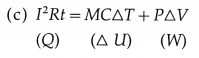Here, I = current through the resistor, R = resistance of the resistor, t = time for which the current is passed through the resistor, M = mass of the water, S = specific heat of water, T = rise in the temperature of water, P = pressure against which the work is done by the water, ∆u= increase in the volume of the water.iii) A mixture of fuel and oxygen is burned in a constant-volume chamber surrounded by a water bath. It was noticed that the temperature of water is increased during the process. Treating the mixture of fuel and oxygen as the system,
(a) Has heat been transferred ?
(b) Has work been done?
(c) What is the sign of ∆u ?
(a) Heat has been transferred from the chamber to the water bath.
(b) No work is done by the system (the mixture of fuel and oxygen) as there is no change in its volume.
(c) There is increase in the temperature of water. Therefore, ∆u is positive for water.
For the system (the mixture of fuel and oxygen), ∆u is negative.

iv) Draw a p-V diagram and explain the concept of positive and negative work. Give one example each.
Consider some quantity of an ideal gas enclosed in a cylinder fitted with a movable, massless and frictionless piston.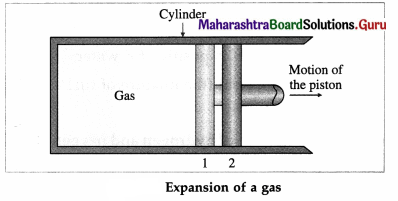Suppose the gas is allowed to expand by moving the piston outward extremely slowly. There is decrease in pressure of the gas as the volume of the gas increases. Below figure shows the corresponding P-V diagram.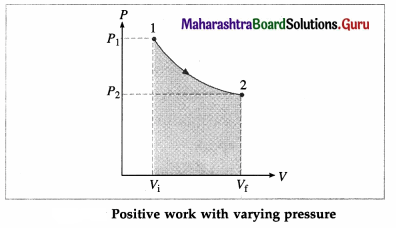In this case, the work done by the gas on its surroundings,
W = $$\int_{V_{\mathrm{i}}}^{V_{\mathrm{f}}} P d V$$ (= area under the curve) is positive as the volume of the gas has increased from Vi to Vf.
Let us now suppose that starting from the same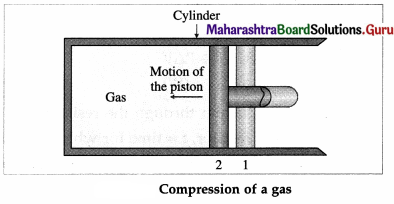initial condition, the piston is moved inward extremely slowly so that the gas is compressed. There is increase in pressure of the gas as the volume of the gas decreases. Figure shows the corresponding P-V diagram.
In this case, the work done by the gas on its surroundings, W = $$\int_{V_{\mathrm{i}}}^{V_{\mathrm{f}}} P d V$$ (= area under the curve) is negative as the volume of the gas has decreased from Vi to Vf.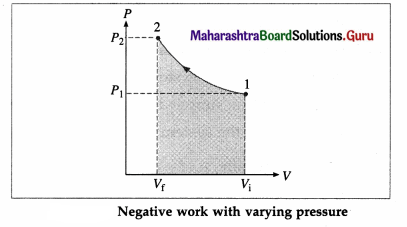v) A solar cooker and a pressure cooker both are used to cook food. Treating them as thermodynamic systems, discuss the similarities and differences between them.
Similarities :

1. Heat is added to the system.
2. There is increase in the internal energy of the system.
3. Work is done by the system on its environment.

Differences : In a solar cooker, heat is supplied in the form of solar radiation. The rate of supply of heat is relatively low.

In a pressure cooker, usually LPG is used (burned) to provide heat. The rate of supply of heat v is relatively high.

As a result, it takes very long time for cooking when a solar cooker is used. With a pressure cooker, it does not take very long time for cooking.
[Note : A solar cooker can be used only when enough solar radiation is available.]

Question 4.
A gas contained in a cylinder fitted with a frictionless piston expands against a constant external pressure of 1 atm from a volume of 5 litres to a volume of 10 litres. In doing so it absorbs 400 J of thermal energy from its surroundings. Determine the change in internal energy of system. [Ans: -106.5 J]
Data : P = 1 atm = 1.013 × 105 Pa, V1 = 5 litres = 5 × 10-3 m3 V2 = 10 litres = 10 × 10-3 m3, Q = 400J.
The work done by the system (gas in this case) on its surroundings,
W = P(V2 – V1)
= (1.013 × 105 Pa) (10 × 10-3 m3 – 5 × 10-3 m3)
= 1.013 (5 × 102)J = 5.065 × 102J
The change in the internal energy of the system, ∆u = Q – W = 400J – 506.5J = -106.5J
The minus sign shows that there is a decrease in the internal energy of the system.

Question 5.
A system releases 130 kJ of heat while 109 kJ of work is done on the system. Calculate the change in internal energy.
[Ans: ∆U = 21 kJ]
Data : Q = -130kj, W= – 109kJ
∆u = Q – W = – 130kJ – ( – 109kJ)
= (-130 + 104) kJ = – 26 kj.
This is the change (decrease) in the internal energy.

Question 6.
Efficiency of a Carnot cycle is 75%. If temperature of the hot reservoir is 727ºC, calculate the temperature of the cold reservoir. [Ans: 23ºC]
Data : η = 75% = 0.75, TH = (273 + 727) K = 1000 K
η = 1 – $$\frac{T_{\mathrm{C}}}{T_{\mathrm{H}}}$$ ∴ $$\frac{T_{\mathrm{C}}}{T_{\mathrm{H}}}$$ = 1 – η
∴ TC = TC(1 – η) = 1000 K (1 – 0.75)
= 250K = (250 – 273)°C
= -23 °C
This is the temperature of the cold reservoir.Question 7.
A Carnot refrigerator operates between 250K and 300K. Calculate its coefficient of performance. [Ans: 5]
Data : TC = 250 K, TH = 300 K
K = $$\frac{T_{\mathrm{C}}}{T_{\mathrm{H}}-T_{\mathrm{C}}}$$ = $$\frac{250 \mathrm{~K}}{300 \mathrm{~K}-250 \mathrm{~K}}$$ = $$\frac{250}{50}$$ = 5
This is the coefficient of performance of the refrigerator.

Question 8.
An ideal gas is taken through an isothermal process. If it does 2000 J of work on its environment, how much heat is added to it? [Ans: 2000J]
Data : W = 2000 J, isothermal process
In this case, the change in the internal energy of the gas, ∆u, is zero as the gas is taken through an isothermal process.
Hence, the heat added to it,
Q = ∆ u + W = 0 + W = 200J

Question 9.
An ideal monatomic gas is adiabatically compressed so that its final temperature is twice its initial temperature. What is the ratio of the final pressure to its initial pressure? [Ans: 5.656]
Data : Tf = 2Ti, monatomic gas ∴ γ = 5/3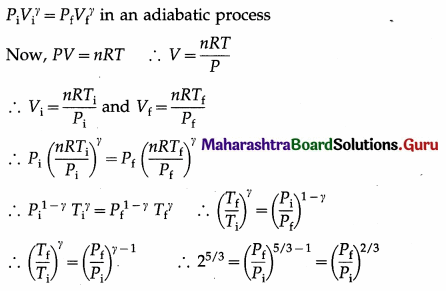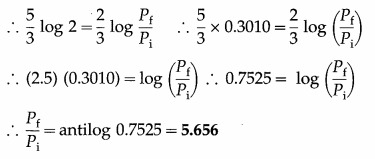This is the ratio of the final pressure (Pf) to the initial pressure (Pi).

Question 10.
A hypothetical thermodynamic cycle is shown in the figure. Calculate the work done in 25 cycles.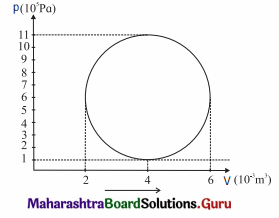[Ans: 7.855 × 104 J]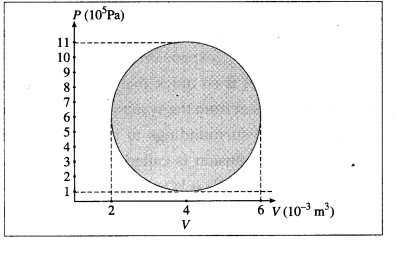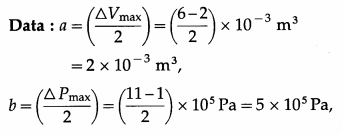25 cycles
The work done in one cycle, $$\oint$$PdV
= πab = (3.142) (2 × 10-3 m3) (5 × 105 Pa)
= 3.142 × 103J
Hence, the work done in 25 cycles
= (25) (3.142 × 103 J) = 7.855 × 104J

Question 11.
The figure shows the V-T diagram for one cycle of a hypothetical heat engine which uses the ideal gas. Draw the p-V diagram and P-V diagram of the system. [Ans: (a)]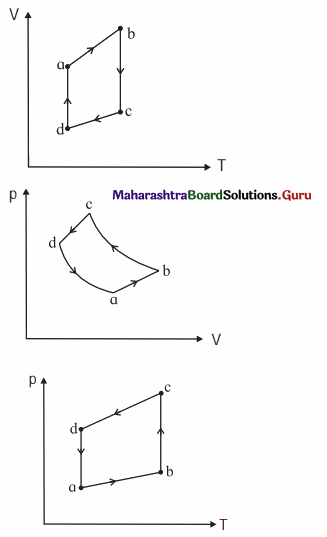[Ans: (b)]
(a) P-V diagram (Schematic)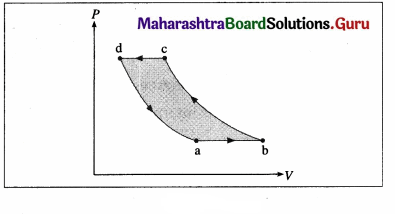ab: isobaric process,
bc : isothermal process,
cd : isobaric process,
da : isothermal process
$$\frac{P_{\mathrm{a}} V_{\mathrm{a}}}{T_{\mathrm{a}}}$$ = $$\frac{P_{\mathrm{b}} V_{\mathrm{b}}}{T_{\mathrm{b}}}$$ = $$\frac{P_{\mathrm{c}} V_{\mathrm{c}}}{T_{\mathrm{c}}}$$ = $$\frac{P_{\mathrm{d}} V_{\mathrm{d}}}{T_{\mathrm{d}}}$$ = nR

(b) P—T diagram (Schematic)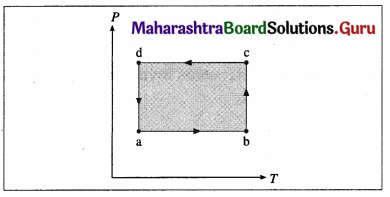Question 12.
A system is taken to its final state from initial state in hypothetical paths as shown figure. Calculate the work done in each case.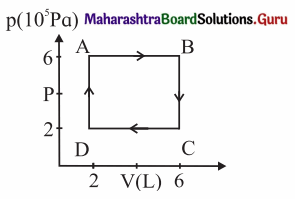[Ans: AB = 2.4 × 106 J, CD = -8 × 105 J, BC and DA zero, because constant volume change]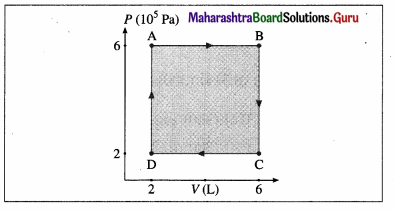Data: PA = PB = 6 × 105 Pa, PC = PD = 2 × 105 Pa VA = VD = 2 L, VB = VC6 L, 1 L = 10-3m3
(i) The work done along the path A → B (isobaric process), WAB = PA (VB – VA) = (6 × 105 Pa)(6 – 2)(10-3 m3) = 2.4 × 103 J
(ii) WBC = zero as the process is isochoric (V = constant).
(iii) The work done along the path C → D (isobaric process), WCD = PC (VD – VC)
= (2 × 105 Pa) (2 – 6) (10-3m3) = -8 × 102J
(iv) WDA = zero as V = constant.

12th Physics Digest Chapter 4 Thermodynamics Intext Questions and Answers

Can you tell? (Textbook Page No. 76)

Question 1.
Why is it that different objects kept on a table at room temperature do not exchange heat with the table ?
The objects do exchange heat with the table but there is no net transfer of energy (heat) as the objects and the table are at the same temperature.

Can you tell? (Textbook Page No. 77)

Question 1.
Why is it necessary to make a physical contact between a thermocouple and the object for measuring its temperature ?
For heat transfer to develop thermoemf.

Can you tell? (Textbook Page No. 81)

Question 1.
Can you explain the thermodynamics involved in cooking food using a pressure cooker ?
Basically, heat is supplied by the burning fuel causing increase in the internal energy of the food (system), and the system does some work on its surroundings. In the absence of any data about the components of food and their thermal and chemical properties, we cannot evaluate changes in internal energy and work done.Use your brain power (Textbook Page No. 85)

Question 1.
Verify that the area under the P-V curve has dimensions of work.
Area under the P-V curve is $$\int_{V_{\mathrm{i}}}^{V_{\mathrm{f}}} P d V$$, where P is the pressure and V is the volume.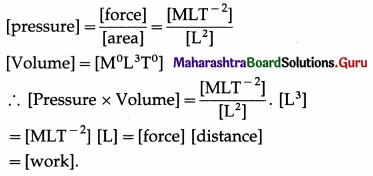Use your brain power (Textbook Page No. 91)

Question 1.
Show that the isothermal work may also be expressed as W = nRT ln$$\left(\frac{\boldsymbol{P}_{\mathrm{i}}}{\boldsymbol{P}_{\mathrm{f}}}\right)$$,
In the usual notation,
W = nRT In $$\frac{V_{\mathrm{f}}}{V_{\mathrm{i}}}$$ and Pi Vi = Pf Vf = nRT in an isothermal process
∴ $$\frac{V_{\mathrm{f}}}{V_{\mathrm{i}}}$$ = $$\frac{P_{\mathrm{i}}}{P_{\mathrm{f}}}$$ and W = nRT ln $$\frac{P_{\mathrm{i}}}{P_{\mathrm{f}}}$$

Use your brain power (Textbook Page No. 94)

Question 1.
Why is the P-V curve for an adiabatic process steeper than that for an isothermal process ?
Adiabatic process : PVγ = constant
∴ VγdP + γPVγ-1 dV = 0
∴ $$\frac{d P}{d V}$$ = – $$\frac{\gamma P}{V}$$
Isothermal process : PV = constant
∴ pdV + VdP = 0 ∴ $$\frac{d P}{d V}$$ = $$\frac{P}{-V}$$
Now, γ > 1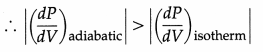∴ $$\frac{d P}{d V}$$ is the slope of the P – V curve.
∴The P – V curve for an adiabatic process is steeper than that for an isothermal process.

Question 2.
Explain formation of clouds at high altitude.
As the temperature of the earth increases due to absorption of solar radiation, water from rivers, lakes, oceans, etc. evaporates and rises to high altitude. Water vapour forms clouds as water molecules come together under appropriate conditions. Clouds are condensed water vapour and are of various type, names as cumulus clouds, nimbostratus clouds, stratus clouds and high-flying cirrus clouds.

Can you tell? (Textbook Page No. 95)

Question 1.
How would you interpret Eq. 4.21 (Q = W) for a cyclic process ?
It means ∆ u = 0 for a cyclic process as the system returns to its initial state.

Question 2.
An engine works at 5000 rpm, and it performs 1000 J of work in one cycle. If the engine runs for 10 min, how much total work is done by the engine ?
The total work done by the engine = (1000 J/cycle) (5000 cycles/min) (10 min) = 5 × 107 J.

Do you know? (Textbook Page No. 101)

Question 1.
Capacity of an air conditioner is expressed in tonne. Do you know why?